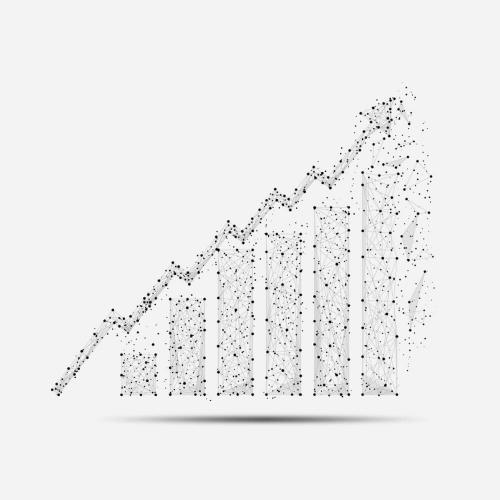# Time Series Analysis

Back to Programs## We created this program for those of you, who are interested in working with time series data.

During the first semester, we focus on the essential fundamentals, such as basic concepts of math and programming, which are the same for all programs.

In the Math & Statistic course, students will learn about discrete mathematics and the basics of probability theory. Algorithms & Data Structures covers the basic algorithms on graphs, data structures, and recurrence relations. Also, during the first semester, students learn how to process and analyze data, and build simple models based on this data in the Data Science course. In the Advanced Programming Languages course, students will learn about programming languages and their fundamental differences.

During the second semester, students will get acquainted with the basics of machine learning, learn how the main machine learning models work (in the Machine Learning course), and also try to use them in practice on complex tasks in the Data Science course (part 2). Students of this program will also take a Systems Architecture training course.

The major course of this program is Practical Time Series Analysis. We will offer students to put into practice their knowledge of algorithms for constructing and analyzing time-series data, including, but not limited to, analysis of various indicators of the financial markets.

## Semester 1

#### Math & Statistics

• Number theory

• Sets, functions, and sequences

• Logic

• Induction

• Sequences

• Combinatorics

• Relations

• Recurrence relations

• Graphs (basics)

• Counting techniques

• Graphs and Numbers

• Growth of Functions

• The probability of an Event

• Conditional probability

• Discrete Random Variables

• Continuous probability

• Sampling Theory

• Statistics

#### Algorithms and Data Structures

• Graphs (basics)

• BFS and DFS

• Dynamic Programming

• LIS, LCS and other 2D problems

• Heaps

• Dijkstra, Floyd, Bellman-Ford

• DFS applications

• Knapsack problem

• Dynamic programming over subsets

• Amortized Time Complexity (Queue via Stack)

• Disjoint Set Union

• Dynamic programming on a tree

• Minimum Spanning Trees

• Matching

• Segment Trees

• Binary Search Trees

• LCA

• What is NP and how is it useful?

• Strings

#### Data Science

• Basic tools for data analysis

• Simple data collection and analysis

• Data Types in Statistics

• Visualization

• Stat in Data Science

• Probability and its applications

• Estimation, Confidence Intervals

• Hypothesis testing

• Correlation and Dependence

• Regression

• Generalized linear model

• Feature engineering (Linear Regression Deep Dive)

• Decision Trees

• ANOVA

• Grammars, Regular Expressions, Backus-Naur Form

• Computation Models

• Functional Programming

• Clojure Programming Language (Basics, Concurrency, Actors, Refs, Atoms, Macros)

• Custom hierarchies and multimethods in Clojure

• Logical Programming

• Prolog

• LLVM (basics, garbage collection)

• Constraint Programming

• MiniZinc

• Guiding the Search in Constraint Programming

• Data Oriented Programming

• Data storage and querying in XML ecosystem

## Semester 2

#### Machine Learning

• Dimensionality Reduction

• Support Vector Machines

• Clustering algorithms

• Perceptrons

• Multi layered perceptrons

• Neural networks for regression and classification problems

• Boosting Algorithms

• Computer vision

• Image processing

• Convolution neural networks (CNNs)

• Object detection

• Reccurent neural networks (RNNs)

#### Systems Architecture

• C++ - syntax, OOP basics, UB, numbers

• RUST - syntax, programming paradigms, safe/unsafe, comparison with C++

• Memory allocation, caching algorithms

• Memory (its components), cache-friendly algorithms

• Assembler (Instructions, RISC, CISC, x86, Intel, asm basics)

• Syscalls

• Processes, Scheduling

• Verilog

• System limits - rlimit, cgoups, Linux namespaces, seccomp

#### Data Science

• Visual data exploration and communication

• Imputation and outlier detection in time series exploratory analytics

• Outlier detection using PyOD library, KNN, SVM and other methods

• Forecasting using neural networks in pytorch

• Data Analysis with Tableau

• Declarative Visualization with Vega-Altair

• Long short-term memory networks (LSTM) for time series

• Recurrent neural networks (RNN) and Gated recurrent units (GRUs) for time series

#### Practical Time Series Analysis

• Introduction to Forecasting

• Baseline models

• Random Walks

• Moving Average Process

• Autoregressive Process

• Forecasting an Autoregressive Process

• Mixed Autoregressive Moving Average Process

• Non-stationary Time Series

• Seasonality

• External Variables

• Forecasting Multiple Time Series

• Introduction to Forecasting with Deep Learning

• Baselines for Deep Learning

• Linear Models

• Implementing a Deep Learning Network

• Long Short-Term Memory Models